Math Calculators, Lessons and Formulas

It is time to solve your math problem

mathportal.org
• Pre algebra
• Decimal numbers
• Decimal approximations

# Decimal approximations

ans:
syntax error
C
DEL
ANS
±
(
)
÷
×
7
8
9
4
5
6
+
1
2
3
=
0
.
auto next question
calculator
•  Question 1: 1 pts Is the following expression true or false? $$384.49 \approx 385$$
•  Question 2: 1 pts Round $2.965$ to the nearest tenth. Use the number line.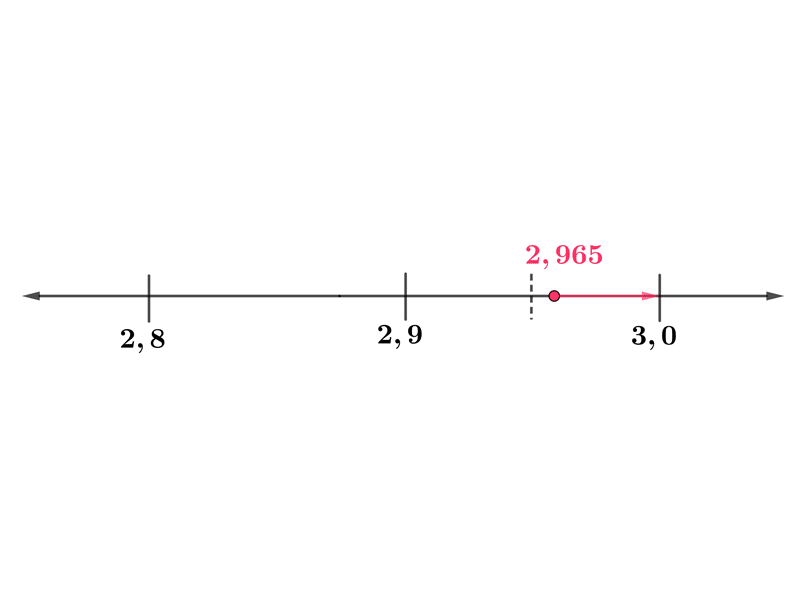$2.8$ $2.9$ $3.0$ $3.4$
•  Question 3: 1 pts Round $4.2231$ to the nearest hundredth. Use the number line.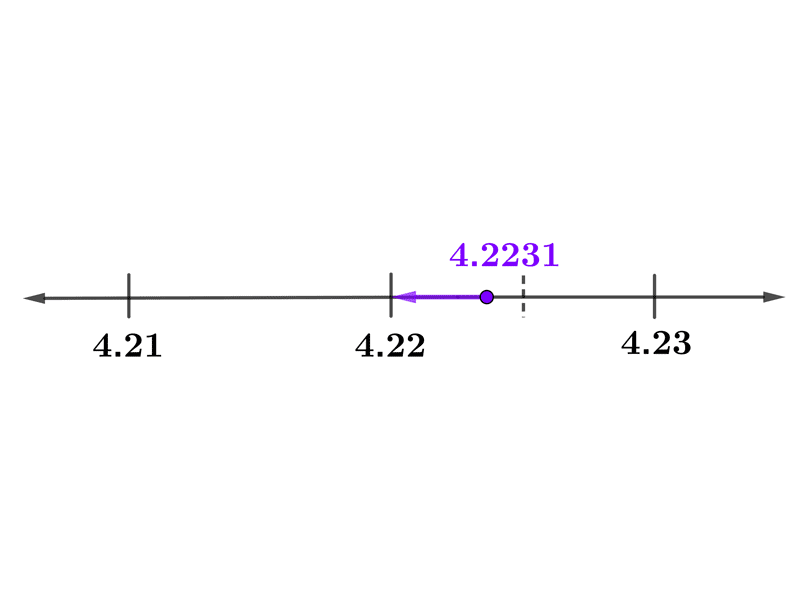$4.21$ $4.22$ $4.23$ $4.24$
•  Question 4: 1 pts Round $4.10354$ to the nearest thousandth. Use the number line.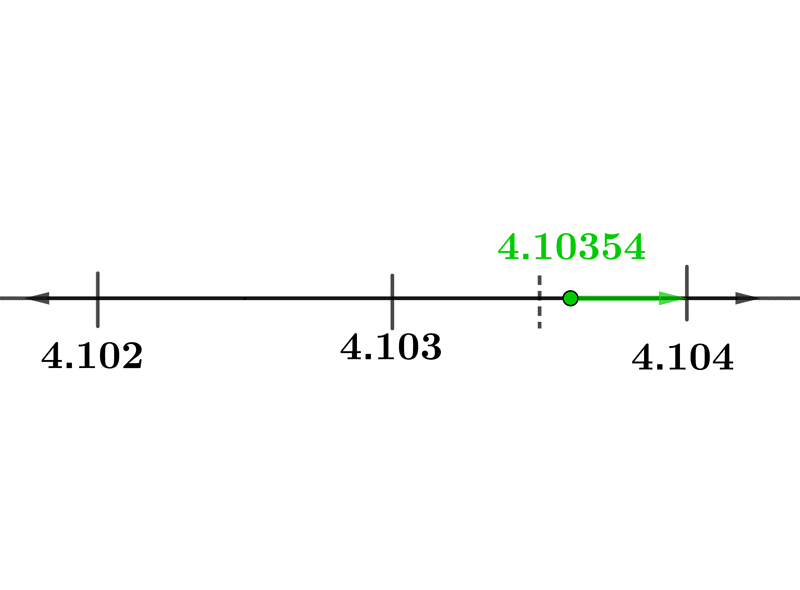$4.102$ $4.103$ $4.104$ $4.105$
•  Question 5: 2 pts Express $1.3267$ to two decimal places.
 $1.31$ $1.32$ $1.33$ $1.34$
•  Question 6: 2 pts Express $0.4663817$ to three decimal places.
 $0.465$ $0.466$ $0.467$ $0.468$
•  Question 7: 2 pts The mass of a ship is stated as 37 659 tonnes to the nearest tonne. Are the following statements correct? The mass is greater than 37659.4 tonnes.
•  Question 8: 2 pts The length of an envelope is $18 cm$ to the nearest $cm$. What is the smallest possible real length of the envelope?
 $16.5$ $17.0$ $17.5$ $17.9$
•  Question 9: 3 pts The interval on the number line below shows all the possible real lengths of the envelope (to the nearest $cm$). Write down the correct numbers for the lower and upper limits of the interval. represented on the diagram by A and B.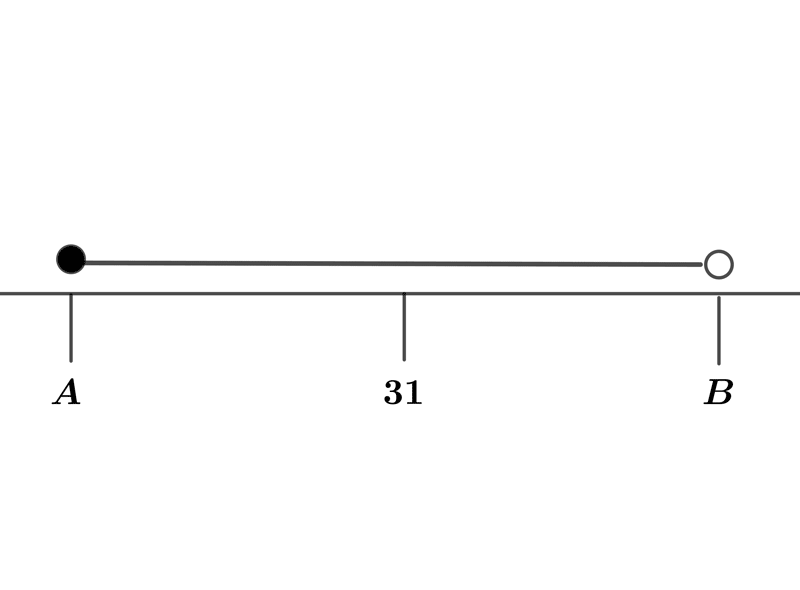$A=30.5; B=31.5$ $A=30.4; B=31.4$ $A=30.0; B=31.0$ $A=30.1; B=31.1$
•  Question 10: 3 pts Write the number 443.9591 correct to 5 significant figures.
 $443.95$ $443.96$ $443.60$ $443.90$
•  Question 11: 3 pts The length of a paper is $18.5 cm$ to the nearest tenth of a $cm.$ The interval on the number line below shows all the possible real lengths of the paper. Write down the numbers represented on the number line by A, B and C.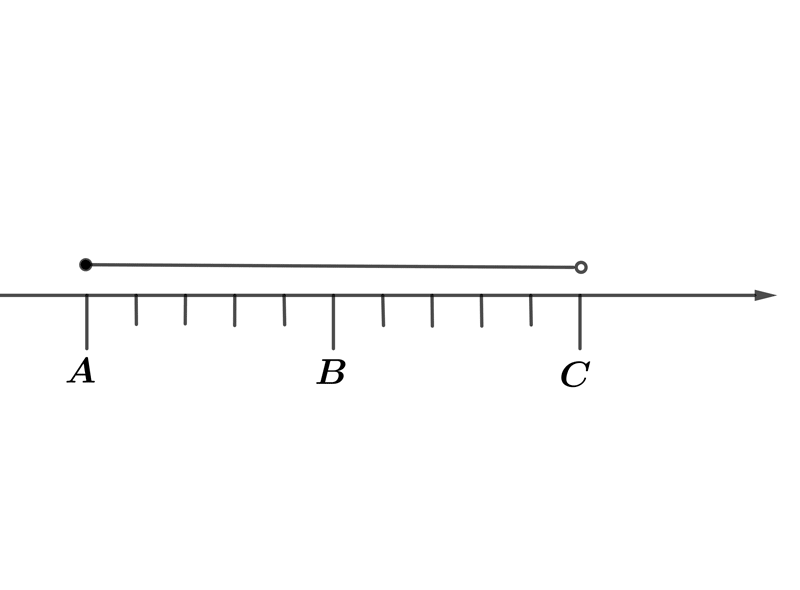$A=18.45; B=18.5; C=19.0$ $A=18.0; B=18.5; C=19.0$ $A=18.49; B=18.5; C=18.51$ $A=18.45; B=18.5; C=18.55$